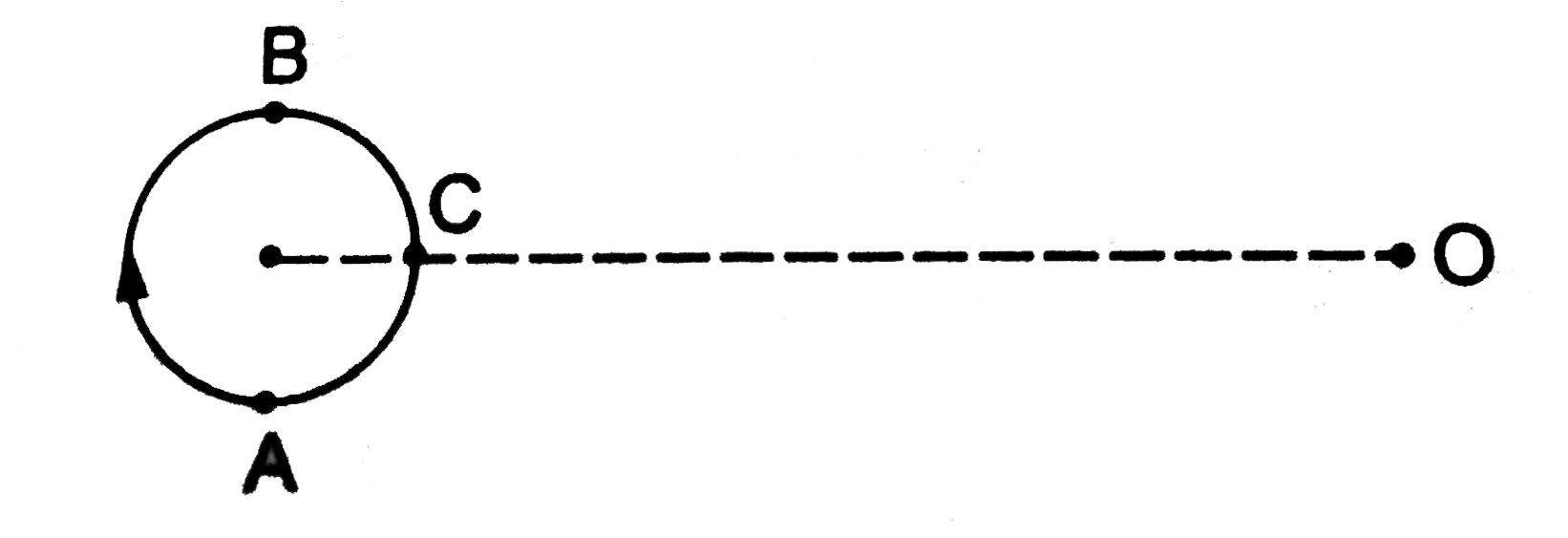# A small source of sound moves on a circle as shown in figure and an observer is sitting at O. Let v_1,v_2,v_3 be the frequencies heard when the sour

33 views
in Physics
closed
A small source of sound moves on a circle as shown in figure and an observer is sitting at O. Let v_1,v_2,v_3 be the frequencies heard when the source is at A,B and C respectively..
A. n_(1)gtn_(2)gtn_(3)
B. n_(2)gtn_(3)gtn_(1)
C. n_(1)=n_(2)=n_(3)
D. n_(2)gtn_(1)gtn_(3)

by (91.5k points)
selected

At point A, source is moving away from observer so apparent frequency n_1 lt n (actual frequency) At point B source is coming towards observer so apparent frequency n_2 gt n and point C source is moving perpendicular to observer so n_3=n.
Hence n_2 lt n_3 gt n_1.